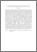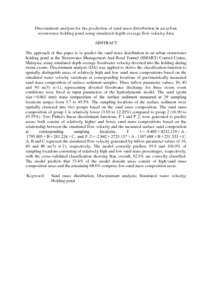# Discriminant analysis for the prediction of sand mass distribution in an urban stormwater holding pond using simulated depth average flow velocity data

## Citation

Dominic, Jeremy Andy and Aris, Ahmad Zaharin and Sulaiman, Wan Nor Azmin and Wan Md Tahir, Wan Zakaria (2016) Discriminant analysis for the prediction of sand mass distribution in an urban stormwater holding pond using simulated depth average flow velocity data. Environmental Monitoring and Assessment, 188 (191). pp. 1-15. ISSN 0167-6369; ESSN: 1573-2959

## Abstract

The approach of this paper is to predict the sand mass distribution in an urban stormwater holding pond at the Stormwater Management And Road Tunnel (SMART) Control Centre, Malaysia, using simulated depth average floodwater velocity diverted into the holding during storm events. Discriminant analysis (DA) was applied to derive the classification function to spatially distinguish areas of relatively high and low sand mass compositions based on the simulated water velocity variations at corresponding locations of gravimetrically measured sand mass composition of surface sediment samples. Three inflow parameter values, 16, 40 and 80 m(3) s(-1), representing diverted floodwater discharge for three storm event conditions were fixed as input parameters of the hydrodynamic model. The sand (grain size > 0.063 mm) mass composition of the surface sediment measured at 29 sampling locations ranges from 3.7 to 45.5%. The sampling locations of the surface sediment were spatially clustered into two groups based on the sand mass composition. The sand mass composition of group 1 is relatively lower (3.69 to 12.20%) compared to group 2 (16.90 to 45.55%). Two Fisher's linear discriminant functions, F 1 and F 2, were generated to predict areas; both consist of relatively higher and lower sand mass compositions based on the relationship between the simulated flow velocity and the measured surface sand composition at corresponding sampling locations. F 1 = -9.405 + 4232.119 × A - 1795.805 × B + 281.224 × C, and F 2 = -2.842 + 2725.137 × A - 1307.688 × B + 231.353 × C. A, B and C represent the simulated flow velocity generated by inflow parameter values of 16, 40 and 80 m(3) s(-1), respectively. The model correctly predicts 88.9 and 100.0% of sampling locations consisting of relatively high and low sand mass percentages, respectively, with the cross-validated classification showing that, overall, 82.8% are correctly classified. The model predicts that 31.4% of the model domain areas consist of high-sand mass composition areas and the remaining 68.6% comprise low-sand mass composition areas.Preview
PDF (Abstract)
Discriminant analysis for the prediction of sand mass distribution in an urban stormwater holding pond using simulated depth average flow velocity data.pdfView Item